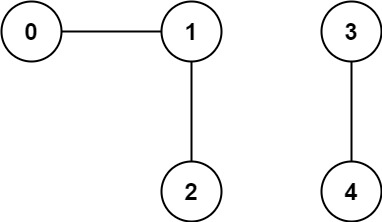# 323. Number of Connected Components in an Undirected Graph

Number of Connected Components in an Undirected Graph - LeetCode You have a graph of n nodes. You are given an integer n and an array edges where edges[i] = [ai, bi] indicates that there is an edge between ai and bi in the graph. Return the number of connected components in the graph.

Example 1:Input: n = 5, edges = [[0,1],[1,2],[3,4]] Output: 2

• code
``````class Solution:
def countComponents(self, n: int, edges: List[List[int]]) -> int:
root = [i for i in range(n)]
rank =  * n
self.valid_union_count = 0

def find(x):
if x == root[x]:
return x
root[x] = find(root[x])
return root[x]

def union(x, y):
rtx = find(x)
rty = find(y)
if rtx == rty:
return
if rank[x] > rank[y]:
root[rty] = rtx
elif rank[x] < rank[y]:
root[rtx] = rty
else:
root[rty] = rtx
rank[rtx] += 1
self.valid_union_count += 1

for x, y in edges:
union(x, y)

return n - self.valid_union_count
``````